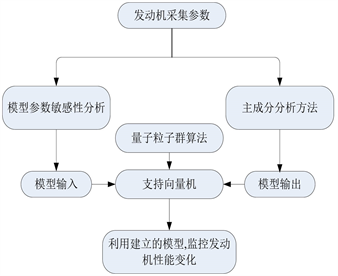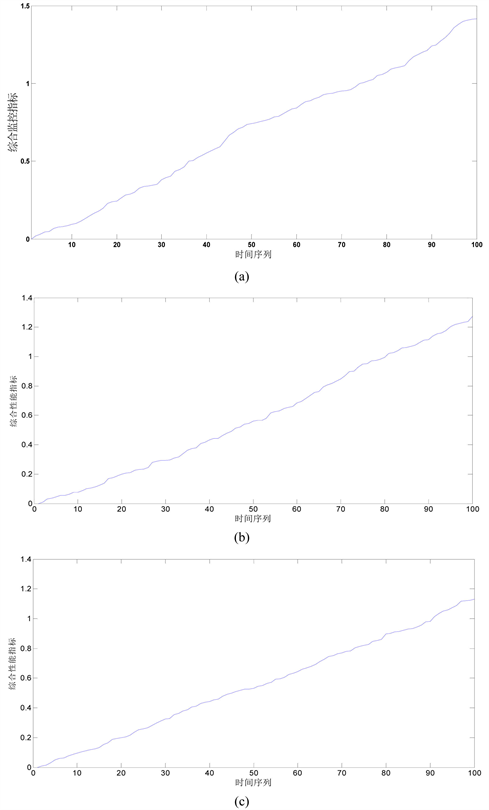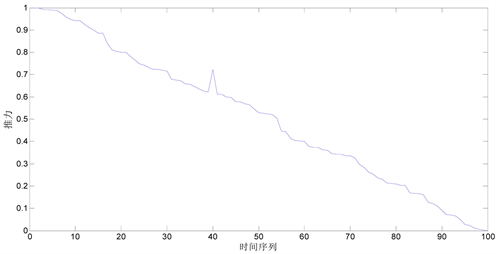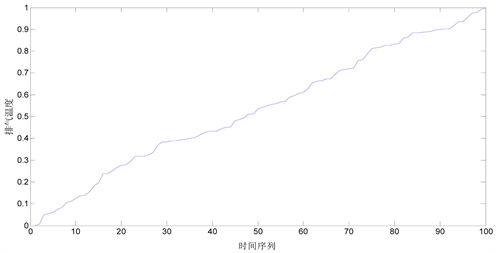﻿ 基于量子粒子群和支持向量机的航空发动机性能监控研究

# 基于量子粒子群和支持向量机的航空发动机性能监控研究Research of Aero-Engine Performance Monitor Based on Quantum Particle Swarm and Support Vector Machine

Abstract: The best aeroengine performance monitor model was established by quantum particle swarm algorithm optimizing key parameters (kernel function parameter σ, insensitive loss coefficient ε, punishment coefficient C) of Support Vector Machine. Thrust and outlet exhaust gas temperature were used as key monitor parameter, and synthetic monitor index was established using Principle Component Analysis on the basis of them. The most concerned parameter was used to model input based on analysis of model sensitivity. The example indicates that established model can monitor engine performance, which provided engine maintenance.

1. 引言

2. 支持向量机基础理论

$y=w\cdot \phi \left(x\right)+b$ (1)

$\begin{array}{l}\underset{w,b,\zeta }{\mathrm{min}}\text{\hspace{0.17em}}\text{\hspace{0.17em}}\text{\hspace{0.17em}}\frac{1}{2}{‖w‖}^{2}+C\underset{i=1}{\overset{n}{\sum }}\left({\zeta }_{i}+{\zeta }_{i}^{2}\right)\\ \text{s}\text{.t}\text{.}\text{\hspace{0.17em}}\text{\hspace{0.17em}}\text{\hspace{0.17em}}\text{ }\text{\hspace{0.17em}}{y}_{i}-w\varphi \left({x}_{i}\right)-b\le \epsilon +{\zeta }_{i}\\ \text{\hspace{0.17em}}\text{\hspace{0.17em}}\text{\hspace{0.17em}}\text{\hspace{0.17em}}\text{\hspace{0.17em}}\text{\hspace{0.17em}}\text{\hspace{0.17em}}\text{\hspace{0.17em}}w\cdot \varphi \left({x}_{i}\right)+b-{y}_{i}\le \epsilon +{\zeta }_{i}^{\ast }\\ \text{\hspace{0.17em}}\text{\hspace{0.17em}}\text{\hspace{0.17em}}\text{\hspace{0.17em}}\text{\hspace{0.17em}}\text{\hspace{0.17em}}\text{\hspace{0.17em}}\text{\hspace{0.17em}}{\zeta }_{i,}{\zeta }_{i}^{\ast }\ge 0,i=1,2,\cdots ,n\end{array}$ (2)

$\begin{array}{l}\mathrm{max}\text{\hspace{0.17em}}\left\{-\frac{1}{2}\underset{i=1}{\overset{n}{\sum }}\underset{j=1}{\overset{n}{\sum }}\left({a}_{i}-{a}_{i}^{\ast }\right)\left({a}_{j}-{a}_{j}^{\ast }\right)×K\left({x}_{i},{x}_{j}\right)-\epsilon \underset{j=1}{\overset{n}{\sum }}\left({a}_{j}+{a}_{j}^{\ast }\right)+\underset{i=1}{\overset{n}{\sum }}{y}_{i}\left({a}_{i}-{a}_{i}^{\ast }\right)\right\}\\ \text{s}\text{.t}\text{.}\text{\hspace{0.17em}}\text{\hspace{0.17em}}\text{\hspace{0.17em}}\underset{i=1}{\overset{}{\sum }}\left({a}_{i}-{a}_{i}^{\ast }\right)=0\\ \text{\hspace{0.17em}}\text{\hspace{0.17em}}\text{\hspace{0.17em}}\text{\hspace{0.17em}}\text{\hspace{0.17em}}\text{\hspace{0.17em}}\text{\hspace{0.17em}}0\le {a}_{i},{a}_{i}^{\ast }\le C\end{array}$ (3)

$K\left({x}_{i},{x}_{j}\right)=\mathrm{exp}\left(\frac{-{\left({x}_{i}-{x}_{j}\right)}^{2}}{{\sigma }^{2}}\right)$ (4)

$y=w\cdot \varphi \left(x\right)+b=\underset{{x}_{i}\in SV}{\sum }\left({a}_{i}-{a}_{i}^{\ast }\right)K\left({x}_{i},X\right)+b$ (5)

3. 量子粒子群算法

${P}_{i}=\left[|\begin{array}{l}\mathrm{cos}\left({\theta }_{i1}\right)\\ \mathrm{sin}\left({\theta }_{i1}\right)\end{array}|\begin{array}{l}\mathrm{cos}\left({\theta }_{i2}\right)\\ \mathrm{sin}\left({\theta }_{i2}\right)\end{array}|\begin{array}{l}\cdots \\ \cdots \end{array}|\begin{array}{l}\mathrm{cos}\left({\theta }_{ip}\right)\\ \mathrm{sin}\left({\theta }_{ip}\right)\end{array}|\right]$ (6)

${X}_{i\text{c}}^{j}=\frac{1}{2}\left[{b}_{i}\left(1+{\alpha }_{i}^{j}\right)+{a}_{i}\left(1-{\alpha }_{i}^{j}\right)\right]$ (7)

${X}_{i\text{s}}^{j}=\frac{1}{2}\left[{b}_{i}\left(1+{\beta }_{i}^{j}\right)+{a}_{i}\left(1-{\beta }_{i}^{j}\right)\right]$ (8)

$\Delta {\theta }_{ij}\left(t+1\right)=w\Delta {\theta }_{ij}\left(t\right)+{c}_{1}{r}_{1}\left(\Delta {\theta }_{l}\right)+{c}_{2}{r}_{2}\left(\Delta {\theta }_{g}\right)$ (9)

$\begin{array}{l}\left[\begin{array}{c}\mathrm{cos}\left({\theta }_{ij}\left(t+1\right)\right)\\ \mathrm{sin}\left({\theta }_{ij}\left(t+1\right)\right)\end{array}\right]=\left[\begin{array}{cc}\mathrm{cos}\left(\Delta {\theta }_{ij}\left(t+1\right)\right)& -\mathrm{sin}\left(\Delta {\theta }_{ij}\left(t+1\right)\right)\\ \mathrm{sin}\left(\Delta {\theta }_{ij}\left(t+1\right)\right)& \mathrm{cos}\left(\Delta {\theta }_{ij}\left(t+1\right)\right)\end{array}\right]\\ \left[\begin{array}{c}\mathrm{cos}\left({\theta }_{ij}\left(t\right)\right)\\ \mathrm{sin}\left({\theta }_{ij}\left(t\right)\right)\end{array}\right]=\left[\begin{array}{c}\mathrm{cos}\left({\theta }_{ij}\left(t\right)+\Delta {\theta }_{ij}\left(t+1\right)\right)\\ \mathrm{sin}\left({\theta }_{ij}\left(t\right)+\Delta {\theta }_{ij}\left(t+1\right)\right)\end{array}\right]\end{array}$ (10)

$\left[\begin{array}{cc}0& 1\\ 1& 0\end{array}\right]\left[\begin{array}{c}\mathrm{cos}\left({\theta }_{ij}\right)\\ \mathrm{sin}\left({\theta }_{ij}\right)\end{array}\right]=\left[\begin{array}{c}\mathrm{sin}\left({\theta }_{ij}\right)\\ \mathrm{cos}\left({\theta }_{ij}\right)\end{array}\right]=\left[\begin{array}{c}\mathrm{cos}\left(\frac{\text{π}}{2}-{\theta }_{ij}\right)\\ \mathrm{sin}\left(\frac{\text{π}}{2}-{\theta }_{ij}\right)\end{array}\right]$ (11)

4. 发动机监控模型建立步骤Figure 1. Monitor model of aeroengine

5. 应用实例

$F=\frac{\mathrm{max}\left(F\right)-F}{\mathrm{max}\left(F\right)-\mathrm{min}\left(F\right)}$

$T=\frac{T-\mathrm{min}\left(T\right)}{\mathrm{max}\left(T\right)-\mathrm{min}\left(T\right)}$ (12)Table 1. Variation of other parameters with change 1% of thrust and exhaust gas temperatureFigure 2. Change of synthetic indexFigure 3. Change of thrustFigure 4. Change of exhaust gas temperature

6. 总结

1) 以支持向量机算法作为发动机监控模型，利用量子粒子群算法确定最佳的模型参数，能够得到最佳的监控模型；

2) 利用模型参数敏感性分析，确定最佳的监控模型输出，减少了模型输入量，提高了监控效率；

3) 以主成分分析的方法，将推力和尾喷口排气温度两个变量转化为单个监控量，第一主成分占到99.44%，以第一主成分作为监控指标，并能够多指标意义相悖的情况，便于更为直观地了解发动机性能状况。并且，监控指标越大，性能下降越严重，与实际分析相符；

4) 本文建立的监控方法，相比少输入参数情况和神经网络监控模型，具有一定的优势，能够真实反映发动机性能变化，反映在曲线上，综合性能指标变化较大。

 李冬, 李本威, 赵鹏飞, 等. 基于卡尔曼滤波和主元分析的发动机性能衰退研究[J]. 海军航空工程学院学报, 2016, 28(2): 127-132.

 马力, 李冬, 薛庆增, 等. 基于量子粒子群和流形学习的分类方法及其在发动机故障诊断中的应用[J]. 推进技术, 2016, 35(10): 1412-1418.

 段中兴, 嵇启春. 催化剂粉尘浓度软测量建模研究与应用[J]. 系统仿真学报, 2008, 20(14): 3899-3902, 3906.

 张一凡, 付忠广. 基于BP神经网络和SVM的电厂粉尘浓度在线监测[J]. 电力与能源进展, 2016, 4(4): 95-102.

 靳涛. 火电机组反向建模方法研究[D]: [博士学位论文]. 北京: 华北电力大学(北京), 2011: 20-27.

 姜艳梅, 卜庆凯. 基于数据挖掘的超市商品销售预测[J]. 数据挖掘, 2018, 8(2): 74-78.

 李景南, 任开春, 余佳玲, 等. 基于活跃目标点粒子群算法的SVM参数选择[J]. 人工智能与机器人研究, 2014(3): 19-24.

 王星博. 主燃油控制系统部件参数对某型发动机气路性能研究[D]: [博士学位论文] 烟台: 海军航空工程学院, 2012.

 李冬, 李本威, 杨欣毅, 朱飞翔. 自适应核模式分析方法及其在航空发动机部件性能衰退识别中的应用[J]. 推进技术, 2013, 34(9): 1272-1278.

 Gomez, G. and Len-dasse, A. (2000) Statistical Fault Isolation with Principal Component Analysis. IFAC Safe Process.

Top